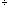My name is Jesus and I am a student having trouble solving this exercise: If we divide the number 1111...........1( it has got 2004 digits) by 3. How many zeros will we get?. The answer that I have found in the book is 667 but I do not know how to reached it. Could you help me please?. Many thanks Hi Jesus, Let me try this problem with 25 1's rather than 2004. 1111111111111111111111111 divided by 3 I did this, longhand and got 11111111111111111111111113 = 0370370370370370370370370 (I wrote the leading zero to help you see the pattern.) I count 9 zeros, but we usually don't count the leading zero, hence I would say that 1111111111111111111111111 divided by 3 has 8 zeros. How can you tell there are 8 zeros without counting? The pattern 037 is three digits long and and 11111111111111111111111111 is 25 digits long. 253 = 8 with a remainder of 1 so in the division, the pattern 037 repeats 8 times and then there is one more digit. Since the pattern starts with a zero there is one more zero. Thus there are 9 zeros, the same as we found by counting, but we don't count the leading zero. Penny